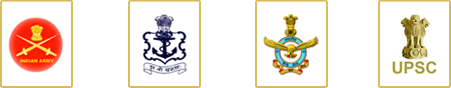### 8. Statistics and Probability

Statistics: Classification of data, Frequency distribution, cumulative frequency distribution – examples Graphical representation – Histogram, Pie Chart, Frequency Polygon – examples. Measures of Central tendency – mean, median and mode. Variance and standard deviation – determination and comparison. Correlation and regression.

### Important Information